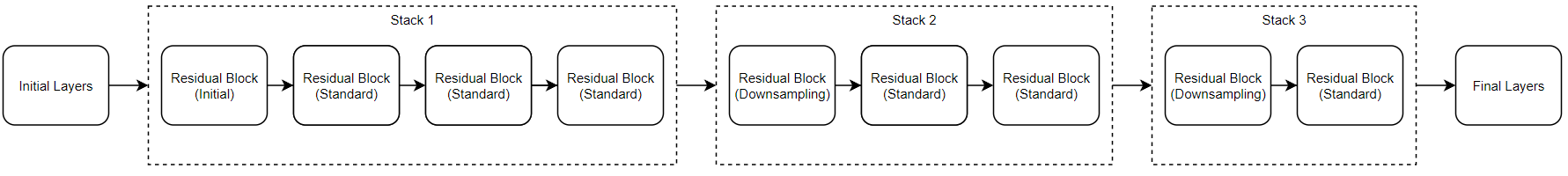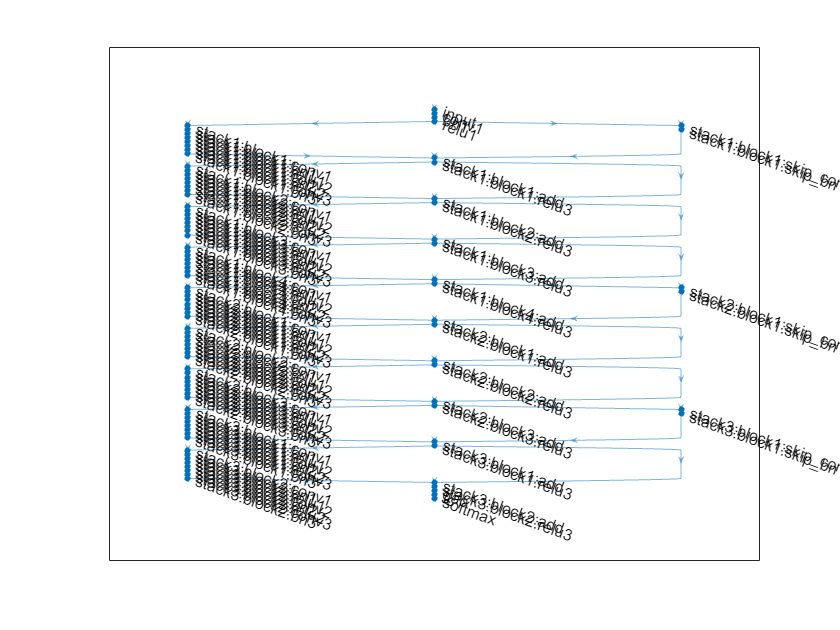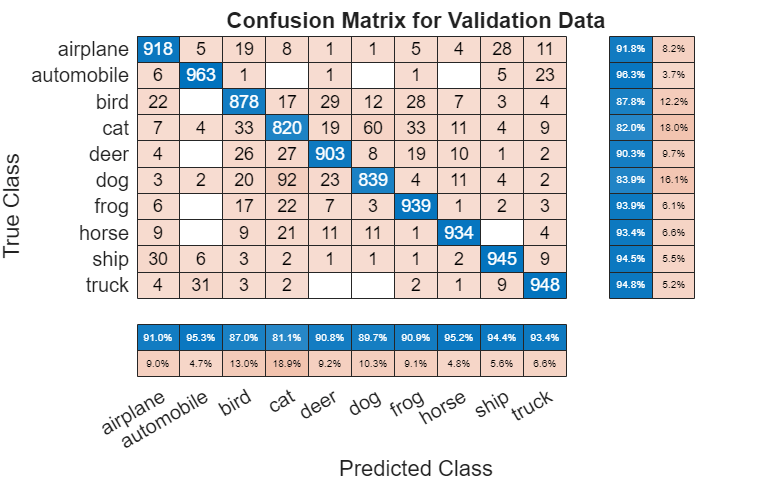# Train Residual Network for Image Classification

This example shows how to create a deep learning neural network with residual connections and train it on CIFAR-10 data. Residual connections are a popular element in convolutional neural network architectures. Using residual connections improves gradient flow through the network and enables training of deeper networks.

For many applications, using a network that consists of a simple sequence of layers is sufficient. However, some applications require networks with a more complex graph structure in which layers can have inputs from multiple layers and outputs to multiple layers. These types of networks are often called directed acyclic graph (DAG) networks. A residual network is a type of DAG network that has residual (or shortcut) connections that bypass the main network layers. Residual connections enable the parameter gradients to propagate more easily from the output layer to the earlier layers of the network, which makes it possible to train deeper networks. This increased network depth can result in higher accuracies on more difficult tasks.

To create and train a network with a graph structure, follow these steps.

• Create a `LayerGraph` object using `layerGraph`. The layer graph specifies the network architecture. You can create an empty layer graph and then add layers to it. You can also create a layer graph directly from an array of network layers. In this case, `layerGraph` connects the layers in the array one after the other.

• Add layers to the layer graph using `addLayers`, and remove layers from the graph using `removeLayers`.

• Connect layers to other layers using `connectLayers`, and disconnect layers from other layers using `disconnectLayers`.

• Plot the network architecture using `plot`.

• Train the network using `trainNetwork`. The trained network is a `DAGNetwork` object.

• Perform classification and prediction on new data using `classify` and `predict`.

You can also load pretrained networks for image classification. For more information, see Pretrained Deep Neural Networks.

### Prepare Data

Download the CIFAR-10 data set . The data set contains 60,000 images. Each image is 32-by-32 in size and has three color channels (RGB). The size of the data set is 175 MB. Depending on your internet connection, the download process can take time.

```datadir = tempdir; downloadCIFARData(datadir);```

Load the CIFAR-10 training and test images as 4-D arrays. The training set contains 50,000 images and the test set contains 10,000 images. Use the CIFAR-10 test images for network validation.

`[XTrain,YTrain,XValidation,YValidation] = loadCIFARData(datadir);`

You can display a random sample of the training images using the following code.

```figure; idx = randperm(size(XTrain,4),20); im = imtile(XTrain(:,:,:,idx),'ThumbnailSize',[96,96]); imshow(im) ```

Create an `augmentedImageDatastore` object to use for network training. During training, the datastore randomly flips the training images along the vertical axis and randomly translates them up to four pixels horizontally and vertically. Data augmentation helps prevent the network from overfitting and memorizing the exact details of the training images.

```imageSize = [32 32 3]; pixelRange = [-4 4]; imageAugmenter = imageDataAugmenter( ... 'RandXReflection',true, ... 'RandXTranslation',pixelRange, ... 'RandYTranslation',pixelRange); augimdsTrain = augmentedImageDatastore(imageSize,XTrain,YTrain, ... 'DataAugmentation',imageAugmenter, ... 'OutputSizeMode','randcrop');```

### Define Network Architecture

The residual network architecture consists of these components:

• A main branch with convolutional, batch normalization, and ReLU layers connected sequentially.

• Residual connections that bypass the convolutional units of the main branch. The outputs of the residual connections and convolutional units are added element-wise. When the size of the activations changes, the residual connections must also contain 1-by-1 convolutional layers. Residual connections enable the parameter gradients to flow more easily from the output layer to the earlier layers of the network, which makes it possible to train deeper networks.

Create Main Branch

Start by creating the main branch of the network. The main branch contains five sections.

• An initial section containing the image input layer and an initial convolution with activation.

• Three stages of convolutional layers with different feature sizes (32-by-32, 16-by-16, and 8-by-8). Each stage contains N convolutional units. In this part of the example, N = 2. Each convolutional unit contains two 3-by-3 convolutional layers with activations. The `netWidth `parameter is the network width, defined as the number of filters in the convolutional layers in the first stage of the network. The first convolutional units in the second and third stages downsample the spatial dimensions by a factor of two. To keep the amount of computation required in each convolutional layer roughly the same throughout the network, increase the number of filters by a factor of two each time you perform spatial downsampling.

• A final section with global average pooling, fully connected, softmax, and classification layers.

Use `convolutionalUnit(numF,stride,tag)` to create a convolutional unit. `numF` is the number of convolutional filters in each layer, `stride` is the stride of the first convolutional layer of the unit, and `tag` is a character array to prepend to the layer names. The `convolutionalUnit` function is defined at the end of the example.

Give unique names to all the layers. The layers in the convolutional units have names starting with `'SjUk'`, where `j` is the stage index and `k` is the index of the convolutional unit within that stage. For example, `'S2U1'` denotes stage 2, unit 1.

```netWidth = 16; layers = [ imageInputLayer([32 32 3],'Name','input') convolution2dLayer(3,netWidth,'Padding','same','Name','convInp') batchNormalizationLayer('Name','BNInp') reluLayer('Name','reluInp') convolutionalUnit(netWidth,1,'S1U1') additionLayer(2,'Name','add11') reluLayer('Name','relu11') convolutionalUnit(netWidth,1,'S1U2') additionLayer(2,'Name','add12') reluLayer('Name','relu12') convolutionalUnit(2*netWidth,2,'S2U1') additionLayer(2,'Name','add21') reluLayer('Name','relu21') convolutionalUnit(2*netWidth,1,'S2U2') additionLayer(2,'Name','add22') reluLayer('Name','relu22') convolutionalUnit(4*netWidth,2,'S3U1') additionLayer(2,'Name','add31') reluLayer('Name','relu31') convolutionalUnit(4*netWidth,1,'S3U2') additionLayer(2,'Name','add32') reluLayer('Name','relu32') averagePooling2dLayer(8,'Name','globalPool') fullyConnectedLayer(10,'Name','fcFinal') softmaxLayer('Name','softmax') classificationLayer('Name','classoutput') ];```

Create a layer graph from the layer array. `layerGraph` connects all the layers in `layers` sequentially. Plot the layer graph.

```lgraph = layerGraph(layers); figure('Units','normalized','Position',[0.2 0.2 0.6 0.6]); plot(lgraph);```Create Residual Connections

Add residual connections around the convolutional units. Most residual connections perform no operations and simply add element-wise to the outputs of the convolutional units.

Create the residual connection from the `'reluInp'` to the `'add11'` layer. Because you specified the number of inputs to the addition layer to be two when you created the layer, the layer has two inputs with the names `'in1'` and `'in2'`. The final layer of the first convolutional unit is already connected to the `'in1'` input. The addition layer then sums the outputs of the first convolutional unit and the `'reluInp'` layer.

In the same way, connect the `'relu11'` layer to the second input of the` 'add12'` layer. Check that you have connected the layers correctly by plotting the layer graph.

```lgraph = connectLayers(lgraph,'reluInp','add11/in2'); lgraph = connectLayers(lgraph,'relu11','add12/in2'); figure('Units','normalized','Position',[0.2 0.2 0.6 0.6]); plot(lgraph);```When the layer activations in the convolutional units change size (that is, when they are downsampled spatially and upsampled in the channel dimension), the activations in the residual connections must also change size. Change the activation sizes in the residual connections by using a 1-by-1 convolutional layer together with its batch normalization layer.

```skip1 = [ convolution2dLayer(1,2*netWidth,'Stride',2,'Name','skipConv1') batchNormalizationLayer('Name','skipBN1')]; lgraph = addLayers(lgraph,skip1); lgraph = connectLayers(lgraph,'relu12','skipConv1'); lgraph = connectLayers(lgraph,'skipBN1','add21/in2');```

Add the identity connection in the second stage of the network.

`lgraph = connectLayers(lgraph,'relu21','add22/in2');`

Change the activation size in the residual connection between the second and third stages by another 1-by-1 convolutional layer together with its batch normalization layer.

```skip2 = [ convolution2dLayer(1,4*netWidth,'Stride',2,'Name','skipConv2') batchNormalizationLayer('Name','skipBN2')]; lgraph = addLayers(lgraph,skip2); lgraph = connectLayers(lgraph,'relu22','skipConv2'); lgraph = connectLayers(lgraph,'skipBN2','add31/in2');```

Add the last identity connection and plot the final layer graph.

```lgraph = connectLayers(lgraph,'relu31','add32/in2'); figure('Units','normalized','Position',[0.2 0.2 0.6 0.6]); plot(lgraph)```### Create Deeper Network

To create a layer graph with residual connections for CIFAR-10 data of arbitrary depth and width, use the supporting function residualCIFARlgraph.

`lgraph = residualCIFARlgraph(netWidth,numUnits,unitType)` creates a layer graph for CIFAR-10 data with residual connections.

• `netWidth` is the network width, defined as the number of filters in the first 3-by-3 convolutional layers of the network.

• `numUnits` is the number of convolutional units in the main branch of network. Because the network consists of three stages where each stage has the same number of convolutional units, `numUnits` must be an integer multiple of 3.

• `unitType` is the type of convolutional unit, specified as `"standard"` or `"bottleneck"`. A standard convolutional unit consists of two 3-by-3 convolutional layers. A bottleneck convolutional unit consists of three convolutional layers: a 1-by-1 layer for downsampling in the channel dimension, a 3-by-3 convolutional layer, and a 1-by-1 layer for upsampling in the channel dimension. Hence, a bottleneck convolutional unit has 50% more convolutional layers than a standard unit, but only half the number of spatial 3-by-3 convolutions. The two unit types have similar computational complexity, but the total number of features propagating in the residual connections is four times larger when using the bottleneck units. The total depth, defined as the maximum number of sequential convolutional and fully connected layers, is 2*`numUnits` + 2 for networks with standard units and 3*`numUnits` + 2 for networks with bottleneck units.

Create a residual network with nine standard convolutional units (three units per stage) and a width of 16. The total network depth is 2*9+2 = 20.

```numUnits = 9; netWidth = 16; lgraph = residualCIFARlgraph(netWidth,numUnits,"standard"); figure('Units','normalized','Position',[0.1 0.1 0.8 0.8]); plot(lgraph)```### Train Network

Specify training options. Train the network for 80 epochs. Select a learning rate that is proportional to the mini-batch size and reduce the learning rate by a factor of 10 after 60 epochs. Validate the network once per epoch using the validation data.

```miniBatchSize = 128; learnRate = 0.1*miniBatchSize/128; valFrequency = floor(size(XTrain,4)/miniBatchSize); options = trainingOptions('sgdm', ... 'InitialLearnRate',learnRate, ... 'MaxEpochs',80, ... 'MiniBatchSize',miniBatchSize, ... 'VerboseFrequency',valFrequency, ... 'Shuffle','every-epoch', ... 'Plots','training-progress', ... 'Verbose',false, ... 'ValidationData',{XValidation,YValidation}, ... 'ValidationFrequency',valFrequency, ... 'LearnRateSchedule','piecewise', ... 'LearnRateDropFactor',0.1, ... 'LearnRateDropPeriod',60);```

To train the network using `trainNetwork`, set the `doTraining` flag to `true`. Otherwise, load a pretrained network. Training the network on a good GPU takes about two hours. If you do not have a GPU, then training takes much longer.

```doTraining = false; if doTraining trainedNet = trainNetwork(augimdsTrain,lgraph,options); else load('CIFARNet-20-16.mat','trainedNet'); end```### Evaluate Trained Network

Calculate the final accuracy of the network on the training set (without data augmentation) and validation set.

```[YValPred,probs] = classify(trainedNet,XValidation); validationError = mean(YValPred ~= YValidation); YTrainPred = classify(trainedNet,XTrain); trainError = mean(YTrainPred ~= YTrain); disp("Training error: " + trainError*100 + "%")```
```Training error: 2.862% ```
`disp("Validation error: " + validationError*100 + "%")`
```Validation error: 9.76% ```

Plot the confusion matrix. Display the precision and recall for each class by using column and row summaries. The network most commonly confuses cats with dogs.

```figure('Units','normalized','Position',[0.2 0.2 0.4 0.4]); cm = confusionchart(YValidation,YValPred); cm.Title = 'Confusion Matrix for Validation Data'; cm.ColumnSummary = 'column-normalized'; cm.RowSummary = 'row-normalized';```You can display a random sample of nine test images together with their predicted classes and the probabilities of those classes using the following code.

```figure idx = randperm(size(XValidation,4),9); for i = 1:numel(idx) subplot(3,3,i) imshow(XValidation(:,:,:,idx(i))); prob = num2str(100*max(probs(idx(i),:)),3); predClass = char(YValPred(idx(i))); title([predClass,', ',prob,'%']) end ```

`convolutionalUnit(numF,stride,tag)` creates an array of layers with two convolutional layers and corresponding batch normalization and ReLU layers. `numF` is the number of convolutional filters, `stride` is the stride of the first convolutional layer, and `tag` is a tag that is prepended to all layer names.

```function layers = convolutionalUnit(numF,stride,tag) layers = [ convolution2dLayer(3,numF,'Padding','same','Stride',stride,'Name',[tag,'conv1']) batchNormalizationLayer('Name',[tag,'BN1']) reluLayer('Name',[tag,'relu1']) convolution2dLayer(3,numF,'Padding','same','Name',[tag,'conv2']) batchNormalizationLayer('Name',[tag,'BN2'])]; end```

 Krizhevsky, Alex. "Learning multiple layers of features from tiny images." (2009). https://www.cs.toronto.edu/~kriz/learning-features-2009-TR.pdf

 He, Kaiming, Xiangyu Zhang, Shaoqing Ren, and Jian Sun. "Deep residual learning for image recognition." In Proceedings of the IEEE conference on computer vision and pattern recognition, pp. 770-778. 2016.